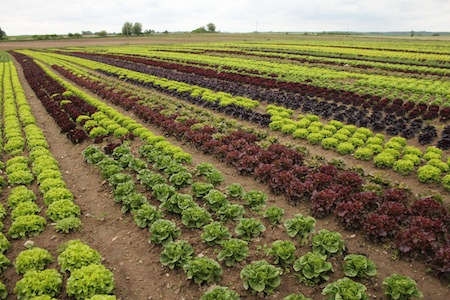## Volt ohne RaumOrganic lettuce in Brandenburg

Here a quick rather handwavy calculation on different area needs for energy production.
This time energy production from humans is compared with photovoltaic electric energy production. There is a tl;dr at the end of the calculation.

Assume Germany would be in a Tropical climate. i.e. the temperature is at least room temperature, so no need for heating and assume thus that the main demands of a human were just food, i.e no car, no cell phone etc.
To make things easy, let’s take the data from this survey from Agree.aua.gr
There the utilized agricultural area for Germany is given in 2007 (p. 13 Table 4) as
\$latex 1693*10^8m^2\$, so if the agricultural land need for a person would be \$latex 1693 m^2\$ then 100 Million people could be fed.
Since 2007 the agricultural land in Germany declined and according to world bank there were in 2012 only about \$latex 1400 m^2\$ agricultural land per person available and Germany has currently roughly only 80 million inhabitants but it’s sort of the same order of magnitude, so let’s take the 2007 numbers. Let’s assume a human is superefficient, i.e. all of the consumed energy via food intake could be reused, just as electric power may be reused.
yes….this is a bit unrealistic but…
Let’s further assume a human needs about \$latex 1500\$ kcal which is \$latex 1.743\$ KWh per day, i.e in a year \$latex 1.743 * 365\$ kWh \$latex = 636.195\$ kWh. So 100 million people need \$latex 63619\$ million KWh. According to table 3 (p.12) the energy need (machines etc.) for agriculture itself is \$latex 42* 10^{15}J\$ which is about 11.6 million KWh. This is not much against the \$latex 63619\$ million KWh, but let’s add it to conclude that the energy need via food intake for 100 million people is roughly \$latex 63630\$ million Kwh. Or by our 100% efficiency assumption the (food production) land need for producing \$latex 63630\$ million Kwh human energy is \$latex 1693*10^8m^2.\$

Let’s compare this with photovoltaic energy. Also if we have assumed tropical temperatures lets assume that the sun still shines dimly as in Germany so let’s take as an average 1500 sunhours per year. A typical solar 120-130 W solar panel is around \$latex 0.8 m^2.\$ So in an hour 1kWh needs thus more than \$latex 100/130*0.8 m^2\simeq 6m^2\$. Let’s take \$latex 7 m^2.\$ So in \$latex 1500\$ sun hours \$latex 7 m^2\$ produce around \$latex 1500 kWh\$ energy.

Hence the land need for producing a solar energy of \$latex 63630\$ million Kwh is only \$latex 63630 \; million\; KWh/1500 KWh *7 m^2 = 3*10^8m^2\$.

Photovoltaic energy production has the big disadvantage that the possibilities for energy storage are still rather bad -but still- the different land need is roughly a factor 560.
Where it has to be said that photovoltaic electric energy production is considered already very area consuming.

tl;dr :
If 100 million humans would rather hyperefficiently convert all food energy in a year into “available energy” , i.e. roughly “produce” \$latex 63630\$ million Kwh then the land need for this is roughly \$latex 1693*10^8m^2.\$
The land need of typical photovoltaic solar cells for this energy in a year is roughly a factor 560 smaller, i.e. \$latex 3*10^8m^2\$.

This calculation is only a very rough estimation, no guarantee for calculation mistakes. I didn’t check thrice, as I would usually do e.g. for an article. So error correction welcome.

supplement 25.10.15: I was actually hesitating wether the energy for the agricultural production should be added, as it is not going to be converted, even not with 100% human conversion efficiency. In principle one would need to compare these agricultural
machine investments with a human labour equivalent (which then would need to be deduced from the converted available human energy – here the 100% efficiency assumption would though beat any machine :)). Similarily I left out any invested energy for food processing (cooking etc.)…it is clear that here it gets even more difficult to let the factor not become bigger, finally it is not so easy for humans to heat up to 100 degrees Celsius.

### 2 Responses to “Volt ohne Raum”

1. SilliKant Walley Says:

Sorry but the assumption of 100% efficiency for a human is quite a bullshit.
As an example: a muscle has a conversion efficiency of 14-27% while an electric motor has up to 99% conversion efficiency!

It may sound cruel, but thats how it is: a robotic lawn mower powered by solar is much cleaner that a human. It’s also cheaper by the way.

2. Rafik Srivanatyam Says:

It may sound cruel, but thats how it is: a robotic lawn mower powered by solar is much cleaner that a human.

I agree -humans are probably the greatest danger for the environment.

I hope they take birth rates into account at the 2015 United Nations Climate Change Conference.

The below box is for leaving comments. Interesting comments in german, french and russian will eventually be translated into english. If you write a comment you consent to our data protection practices as specified here. If your comment text is not too rude and if your URL is not clearly SPAM then both will be published after moderation. Your email adress will not be published. Moderation is done by hand and might take up to a couple of days.
you can use LaTeX in your math comments, by using the [latex] shortcode:
[latex] E = m c^2 [/latex]